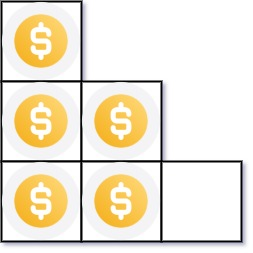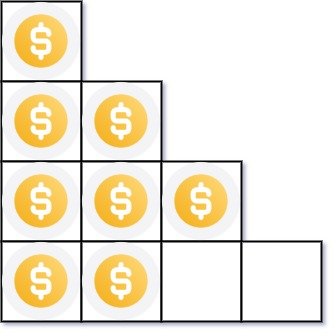### LeetCode• ㊗️
• 大家
• offer
• 多多！

## Problem

You have n coins and you want to build a staircase with these coins. The staircase consists of k rows where the ith row has exactly i coins. The last row of the staircase may be incomplete.

Given the integer n, return the number of complete rows of the staircase you will build.

Example 1:Input: n = 5
Output: 2
Explanation: Because the 3rd row is incomplete, we return 2.


Example 2:Input: n = 8
Output: 3
Explanation: Because the 4th row is incomplete, we return 3.


## Code

class Solution {
public int arrangeCoins(int n) {
return (int)(Math.sqrt(2 * (long)n + 0.25) - 0.5);
}
}